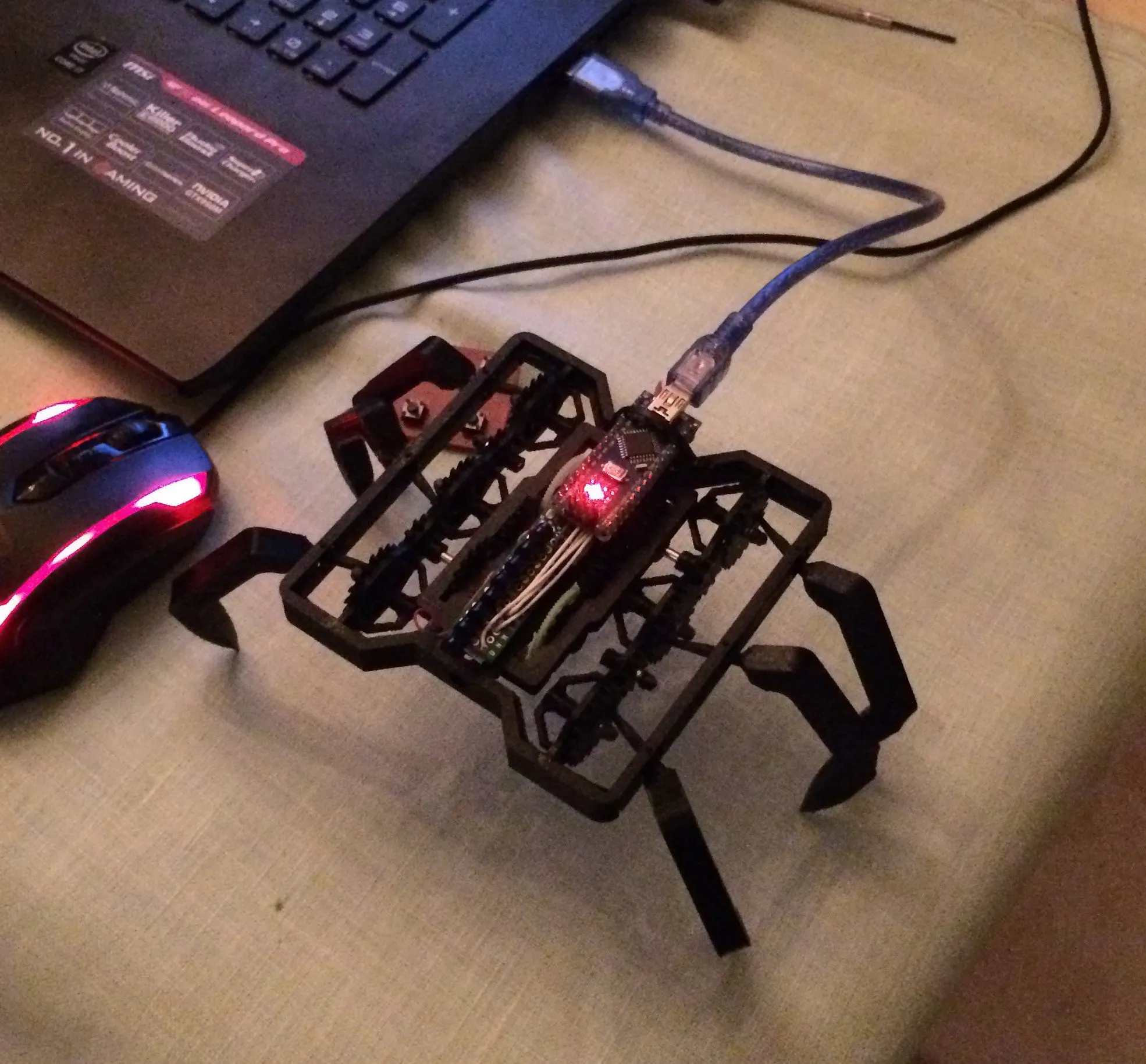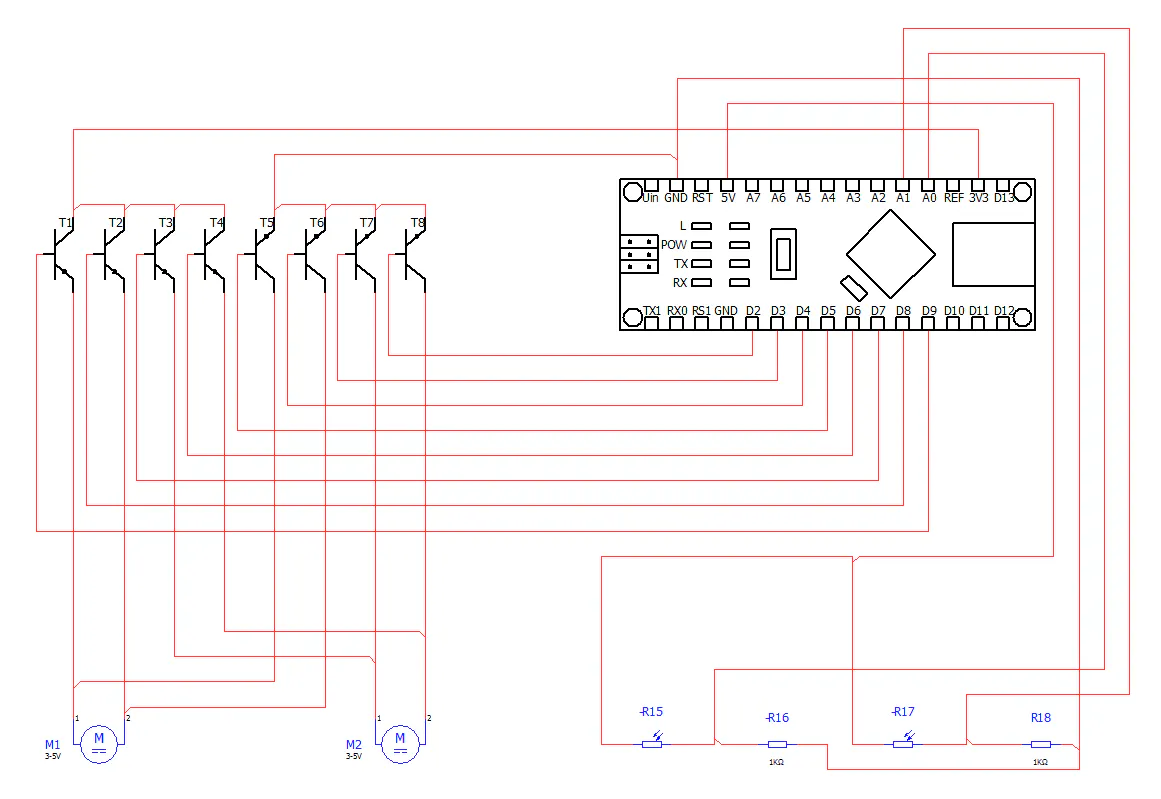# 1 KB 6-Legged Crawling Bot

The robot is controlled with an Arduino Nano and only uses 2 motors and 2 sensors. The program to control the robot is only 843 bytes big.## Things used in this project

### Hardware components

 arduino nano
×1
 small coreless motor from a small rc helikopter
×2
 NPN transistor of the C-type
×8
 pcb prototype board
×1
 antenna for bearings
×2
 photosensor
×2
 1 K ohm resistor
×2
 3D printed parts in PLA plastic
×41
 2s 200mah LIPO battery
×1
 flexible coper wire 0.1-0.5 mm you need about 1 meter
×1
 superglue
×1

## Schematics

### circuit diagram## Code

### 1kb 6 legged crawling robot code

C/C++
```int T1 = 9;
int T2 = 8;
int T3 = 7;
int T4 = 6;
int T5 = 5;
int T6 = 4;
int T7 = 3;
int T8 = 2;
int S1 = A0;
int S2 = A1;
void setup(){
pinMode(T1, OUTPUT);
pinMode(T2, OUTPUT);
pinMode(T3, OUTPUT);
pinMode(T4, OUTPUT);
pinMode(T5, OUTPUT);
pinMode(T6, OUTPUT);
pinMode(T7, OUTPUT);
pinMode(T8, OUTPUT);
pinMode(S1, INPUT);
pinMode(S2, INPUT);
}
void loop(){
if(val1 > val2){
analogWrite(T1, 240);
analogWrite(T6, 255);
analogWrite(T2, 0);
analogWrite(T5, 0);
analogWrite(T3, 240);
analogWrite(T8, 255);
analogWrite(T4, 0);
analogWrite(T7, 0);
}
else{
analogWrite(T1, 240);
analogWrite(T6, 255);
analogWrite(T2, 0);
analogWrite(T5, 0);
analogWrite(T3, 0);
analogWrite(T8, 0);
analogWrite(T4, 240);
analogWrite(T7, 255);
}
}
```

## Credits

### Bribro12

14 projects • 10 followers
I'm a maker. I design and create my own projects and build them from scratch. My favorite tools are my 3D-printer and laser cutter